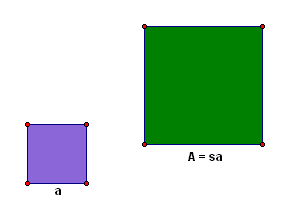﻿ Perimeters and Areas of Similar Polygons
PERIMETERS and AREAS of SIMILAR POLYGONS
by Dr. Carol JVF Burns (website creator)
Follow along with the highlighted text while you listen!

Suppose that a triangle with sides $\,a\,$, $\,b\,$, and $\,c\,$ has been scaled by a positive number $\,s\,$
to get a similar triangle with corresponding sides $\,A\,$, $\,B\,$, and $\,C\,$.

Thus, $\,A = sa\,$ and $\,B = sb\,$ and $\,C = sc\,$.Let's investigate the relationship between the perimeters of these two triangles:

$\text{perimeter of original triangle} = a + b + c\,$

$\text{perimeter of scaled triangle}$
$= A + B + C$
$= sa + sb + sc$
$= s(a + b + c)$
$= s(\text{perimeter of original triangle})$

Thus, the perimeter ends up being scaled by the same factor that scales the sides!

This can be re-phrased in terms of ratios.
Notice that: $$\cssId{s17}{\frac{\text{scaled perimeter}}{\text{original perimeter}}} \cssId{s18}{= \frac{A+B+C}{a+b+c}} \cssId{s19}{= \frac{sa+sb+sc}{a+b+c}} \cssId{s20}{= \frac{s(a+b+c)}{a+b+c}} \cssId{s21}{= s} \cssId{s22}{= \text{scaling factor}}$$ and $$\cssId{s24}{\frac{\text{scaled side}}{\text{original side}}} \cssId{s25}{= \frac{A}{a}} \cssId{s26}{= \frac{sa}{a}} \cssId{s27}{= s}$$ $$\cssId{s28}{\frac{\text{scaled side}}{\text{original side}} = \frac{B}{b} = \frac{sb}{b} = s}$$ $$\cssId{s29}{\frac{\text{scaled side}}{\text{original side}} = \frac{C}{c} = \frac{sc}{c} = s}$$ So, the ratio of the perimeters is the same as the ratio of corresponding sides.

A similar calculation shows that this result is indeed true for polygons in general:

THEOREM Perimeters of Similar Polygons
The ratio of the perimeters of two similar polygons is equal to the ratio of the corresponding sides.

That is: $$\cssId{s36}{\frac{\text{perimeter of scaled polygon}}{\text{perimeter of original polygon}}} \cssId{s37}{= \text{the scaling factor}} \cssId{s38}{= \frac{\text{scaled side}}{\text{original side}}}$$
 Area, on the other hand, behaves quite differently. Suppose you have a square of side $\,a\,$ that has been scaled by $\,s\,$ to get a square of side $\,A\,$. Thus, $\,A = sa\,$. Let's investigate the relationship between the areas of these two squares: $\cssId{s45}{\text{area of original square}} \cssId{s46}{= a\cdot a} \cssId{s47}{= a^2}$ $\text{area of scaled square}$ $= A\cdot A$ $= (sa)\cdot(sa)$ $= s^2\cdot a^2$ $= s^2(\text{area of original square})$ Thus, the area ends up being scaled by the square of the factor that scales the sides!Again, this can be re-phrased in terms of ratios.
Notice that: $$\cssId{s56}{\frac{\text{scaled square area}}{\text{original square area}}} \cssId{s57}{= \frac{A\cdot A}{a \cdot a}} \cssId{s58}{= \frac{(sa)\cdot (sa)}{a\cdot a}} \cssId{s59}{= \frac{s^2a^2}{a^2}} \cssId{s60}{= s^2} \cssId{s61}{= \text{the square of the ratio of corresponding sides}}$$

This idea leads to the following theorem:

THEOREM Areas of Similar Polygons
The ratio of the areas of two similar polygons is equal to the square of the ratio of the corresponding sides.
EXAMPLES:
Question:
Suppose that a triangle has sides of lengths $\,x\,$, $\,y\,$, and $\,z\,$.
The sides of the triangle are scaled by a factor of $\,5\,$ to get a similar triangle.
What is a formula for the perimeter of the new triangle?
Solution:
The perimeter is scaled by the same factor as the sides.
The original perimeter is $\,x+y+z\,$.
The new (scaled) perimeter is $\,5(x+y+z)\,$.
Question:
Suppose that the sides of a polygon are scaled by a factor of $\,3\,$ to get a similar polygon.
This new polygon has area $\,198\,$.
What is the area of the original polygon?
Solution:
The area is scaled by the square of the factor that scales the sides.
Let $\,A\,$ denote the (unknown) original area.
We are told that the new (scaled) area is $\,198\,$, and the scaling factor is $\,3\,$.
So, $\,A\,$ gets scaled by $\,3^2\,$ to give $\,198\,$;   i.e., $\,3^2A = 198\,$.
Solving for $\,A\,$ gives $\,A = \frac{198}{3^2} = 22\,$, so the area of the original polygon is $\,22\,$.
Master the ideas from this section